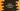# Two ways to find the set difference in Python## Python program to find the difference between two sets :

In this python programming tutorial, we will learn how to find the difference between two sets. The difference between set A and set B is a set that contains only the elements from set A those are not in set B. In this example, we will take the inputs from the user for both sets. The program will calculate the difference and print it out.

## 1. Using difference() method :

For finding out the difference between two sets, python has one inbuilt method difference(). The syntax of the method is as below :

``A.difference(B)``

As you can see, it takes one set as the parameter. It calculates the difference between A and B _i.e. _A - B and returns one new set. Note that this method doesn’t modify the original set.

### Example Program :

``````#1
setA = set()
setB = set()

#2
setA_length = int(input(“Enter the size of the first set : “))
setB_length = int(input(“Enter the size of the second set : “))

#3
print(“\n”)
print(“Enter values for the first set : \n”)
for i in range(setA_length):
e = int(input(“Enter value {} : “.format(i+1)))

#4
print(“\n”)
print(“Enter values for the second set : \n”)
for i in range(setB_length):
e = int(input(“Enter value {} : “.format(i+1)))

#5
print(“\n”)
print(“First set : {}”.format(setA))
print(“Second set : {}”.format(setB))
print(“Difference : {}”.format(setA.difference(setB)))``````

### Explanation :

The commented numbers in the above program denote the step numbers below :

1. Create two empty set setA and setB.
2. Ask the user to enter the size of both sets. Read and store the values.
3. Get the inputs for the first set from the user. Add them all to setA.
4. Similarly, get the inputs and add to the second set setB.
5. Print out the first set, second set and difference between them using the difference method.

### Sample Output :

``````Enter the size of the first set : 3
Enter the size of the second set : 1

Enter values for the first set :

Enter value 1 : 1
Enter value 2 : 2
Enter value 3 : 3

Enter values for the second set :

Enter value 1 : 2

First set : {1, 2, 3}
Second set : {2}
Difference : {1, 3}``````

## 2. Using ‘-‘ operator :

Instead of using the difference() method, we can also use ’-‘ to find out the difference between the two sets.

``````setA = set()
setB = set()

setA_length = int(input(“Enter the size of the first set : “))
setB_length = int(input(“Enter the size of the second set : “))

print(“\n”)
print(“Enter values for the first set : \n”)
for i in range(setA_length):
e = int(input(“Enter value {} : “.format(i+1)))

print(“\n”)
print(“Enter values for the second set : \n”)
for i in range(setB_length):
e = int(input(“Enter value {} : “.format(i+1)))

print(“\n”)
print(“First set : {}”.format(setA))
print(“Second set : {}”.format(setB))
print(“Difference : {}”.format(setA - setB))``````

The above examples are available on Github

### Sample Output :

``````Enter the size of the first set : 4
Enter the size of the second set : 2

Enter values for the first set :

Enter value 1 : 0
Enter value 2 : 1
Enter value 3 : 2
Enter value 4 : 3

Enter values for the second set :

Enter value 1 : 0
Enter value 2 : 3

First set : {0, 1, 2, 3}
Second set : {0, 3}
Difference : {1, 2}``````